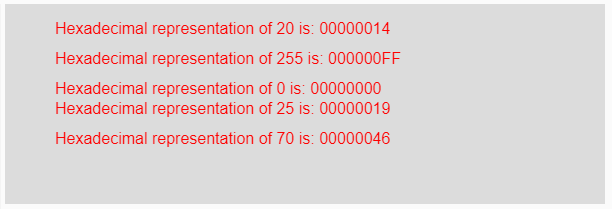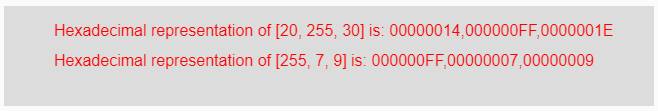# p5.js | hex() function

The hex() function in p5.js is used to convert a number into its hexadecimal notation.

Syntax:

`hex(Number)`

Parameters: This function accepts a parameter Number which are to be converted into its hexadecimal form. This parameter might also be an array of numbers.

Return Value: It returns the converted hexadecimal representation.

Below program illustrates the hex() function in p5.js.
Example-1:

 `function` `setup() { ` ` `  `    ``// Creating Canvas size ` `    ``createCanvas(600, 200); ` `} ` ` `  `function` `draw() { ` ` `  `    ``// Set the background color  ` `    ``background(220); ` ` `  `    ``// Initializing some numbers ` `    ``let Number1 = 20; ` `    ``let Number2 = 255; ` `    ``let Number3 = 0; ` `    ``let Number4 = 25; ` `    ``let Number5 = 70; ` ` `  `    ``// Calling to hex() function. ` `    ``let A = hex(Number1); ` `    ``let B = hex(Number2); ` `    ``let C = hex(Number3); ` `    ``let D = hex(Number4); ` `    ``let E = hex(Number5); ` ` `  `    ``// Set the size of text  ` `    ``textSize(16); ` ` `  `    ``// Set the text color  ` `    ``fill(color(``'red'``)); ` ` `  `    ``// Getting hexadecimal notation ` `    ``text(``"Hexadecimal representation of 20 is: "` `+ A, 50, 30); ` `    ``text(``"Hexadecimal representation of 255 is: "` `+ B, 50, 60); ` `    ``text(``"Hexadecimal representation of 0 is: "` `+ C, 50, 90); ` `    ``text(``"Hexadecimal representation of 25 is: "` `+ D, 50, 110); ` `    ``text(``"Hexadecimal representation of 70 is: "` `+ E, 50, 140); ` `} `

Output:Example-2:

 `function` `setup() { ` ` `  `    ``// Creating Canvas size ` `    ``createCanvas(650, 100); ` `} ` ` `  `function` `draw() { ` ` `  `    ``// Set the background color  ` `    ``background(220); ` ` `  `    ``// Initializing some numbers ` `    ``let Number1 = [20, 255, 30]; ` `    ``let Number2 = [255, 7, 9]; ` ` `  `    ``// Calling to hex() function. ` `    ``let A = hex(Number1); ` `    ``let B = hex(Number2); ` ` `  `    ``// Set the size of text  ` `    ``textSize(16); ` ` `  `    ``// Set the text color  ` `    ``fill(color(``'red'``)); ` ` `  `    ``// Getting hexadecimal notation ` `    ``text(``"Hexadecimal representation of [20, 255, 30] is: "` `     ``+ A, 50, 30); ` `    ``text(``"Hexadecimal representation of [255, 7, 9] is: "`  `     ``+ B, 50, 60); ` `} `

Output:Reference: https://p5js.org/reference/#/p5/hex

My Personal Notes arrow_drop_upCheck out this Author's contributed articles.

If you like GeeksforGeeks and would like to contribute, you can also write an article using contribute.geeksforgeeks.org or mail your article to contribute@geeksforgeeks.org. See your article appearing on the GeeksforGeeks main page and help other Geeks.

Please Improve this article if you find anything incorrect by clicking on the "Improve Article" button below.

Article Tags :

Be the First to upvote.

Please write to us at contribute@geeksforgeeks.org to report any issue with the above content.